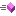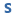﻿ NVector3DF Structure Methods
Nevron .NET Vision
Nevron.GraphicsCore Namespace / NVector3DF Structure

In This Topic
NVector3DF Structure Methods
In This Topic

For a list of all members of this type, see NVector3DF members.

Public Methods
NameDescriptionOverloaded. Makes this vector the vector sum of v1 and v2Makes this vector the nozmalized normal of v1, v2 and v3Makes this vector the non nozmalized normal of v1, v2 and v3Overloaded. Computes the cross product of two 3-D vectorsMakes this vector the cross product of vector A and vector BMakes this vector the scalar division of v1 with the specified fDiv factorOverloaded. Computes the dot product of two 3-D vectorsOverloaded. Determines whether this vector is equal to the specified vectorReturns the hash code of this instance.Gets the vector lengthGets the vector length squareInverts the vectorMakes this vector the scalar multiplication of v1 with the specified fMul factorOverloaded. Computes the normalized version of a 3-D vector.Overloaded.Sets the x and y coordinates of the vectorSets the x, y and z coordinates of the vectorMakes this vector the vector sum of v1 and -v2Overriden to provide a string representation of the vectorDetermines the orientation of three points in the XY plane.Determines the orientation of three points in the XY plane.
Top